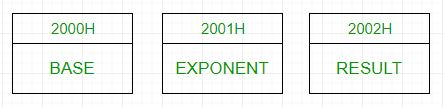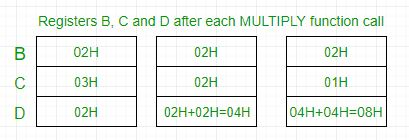# 8085 program to find nth power of a number

Problem – Write an assembly language code for calculating the nth power of a number using 8085 microprocessor.

Example –

```Input : Base=>02H
Exponent=>03H
Output :08H
```In 8085 microprocessor, no direct instruction exists to multiply two numbers, so multiplication is done by repeated addition as 4*4 is equivalent to 4+4+4+4(ie 4 times).
Load 02H(base) to register B and 03H(exponent) to register C -> set D register to 02H -> Add 02H B(ie 2) times -> D register now contains 04H -> Add 04H B(ie 2) times -> D register now contains 08H -> Output is 08H.Algorithm –

1. Load the base into register B and exponent into register C.
2. To start multiplication set D to 01H.
4. Decrements C.
6. Take memory pointer to next location and store result.
7. Load E with contents of B and clear accumulator.
8. Repeatedly add contents of D to accumulator E times.
9. Store accumulator content to D.
10. Go to step 4.

Program –

2000H Base Data Byte for base
2001H Exponent Data Byte for exponent
2002H Result Result of factorial
2003H LXI H, 2000H Load data from memory
2006H MOV B, M Load base to B register
2007H INX H Increment memory
2008H MOV C, M Load exponent to C register
2009H MVI D, 01H Set D register to 1
200BH POWER_LOOP CALL MULTIPLY Subroutine call for multiplication
200EH DCR C Decrement C
200FH JNZ POWER_LOOP Call power_loop till C becomes 0
2012H INX H Increment memory
2013H MOV M, D Store result in memory
2014H HLT Halt
2100H MULTIPLY MOV E, B Transfer contents of B to E
2101H MVI A, 00H Clear accumulator to store result
2104H DCR E Decrement E
2108H MOV D, A Transfer contents of A to D
2109H RET Return from subroutine

Explanation –

1. Set register B with base and register C with exponent.
2. Set register D with base by calling MULTIPLY subroutine one time.
3. Decrement C and add D to itself B times by calling MULTIPLY subroutine.
4. Repeat the above step till C reaches 0 and then exit the program.
5. The result is obtained in D register which is stored in memory

Attention reader! Don’t stop learning now. Get hold of all the important CS Theory concepts for SDE interviews with the CS Theory Course at a student-friendly price and become industry ready.

My Personal Notes arrow_drop_upStudent at Netaji Subhas Institute of Technology

If you like GeeksforGeeks and would like to contribute, you can also write an article using contribute.geeksforgeeks.org or mail your article to contribute@geeksforgeeks.org. See your article appearing on the GeeksforGeeks main page and help other Geeks.

Please Improve this article if you find anything incorrect by clicking on the "Improve Article" button below.

Improved By : aastha98